# UNITER UNiversal Image-TExt Representation Learning

This is my reading note for UNITER: UNiversal Image-TExt Representation Learning. This paper proposes a vision language pre training model. The major innovation here is it studies the work region alignment loss as well as different mask region models task.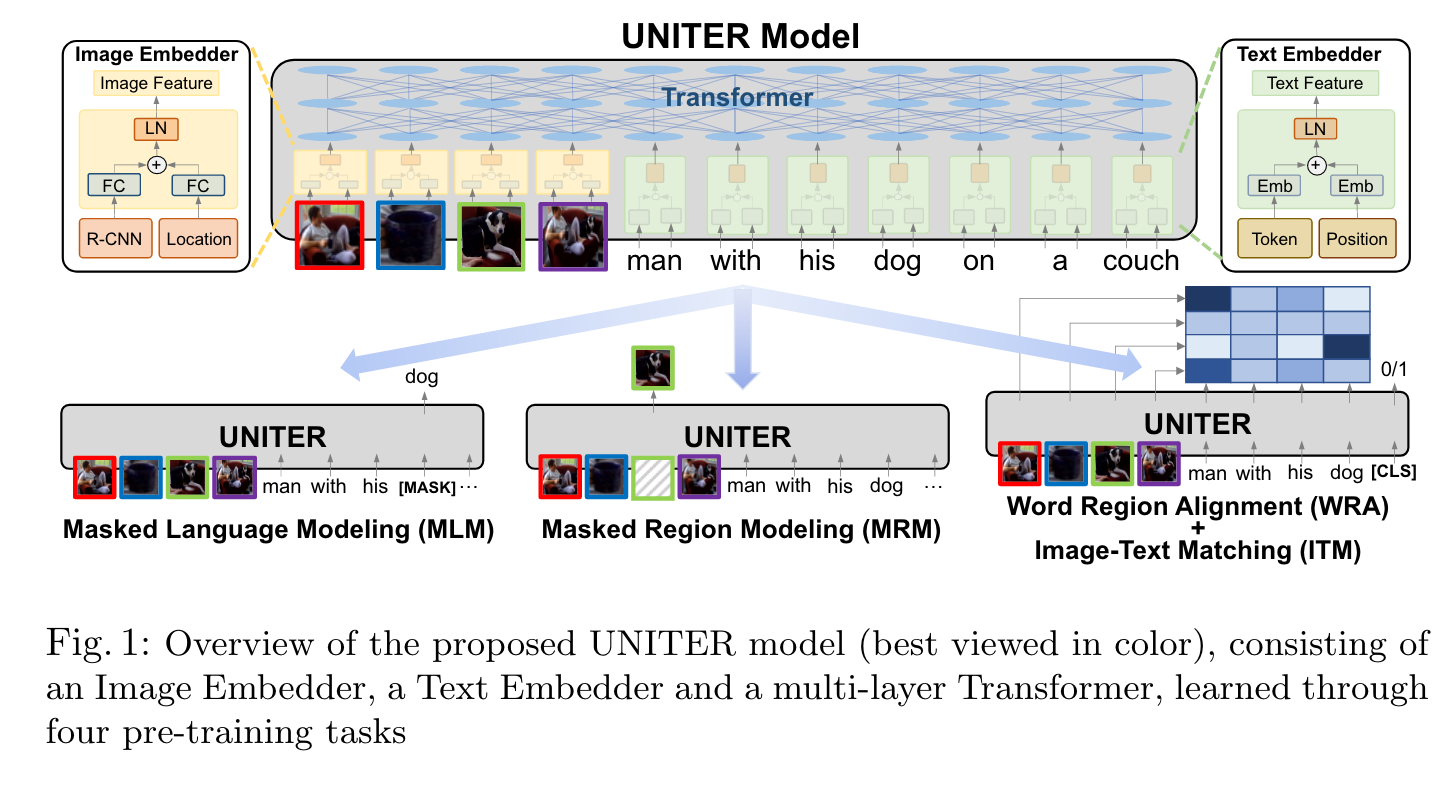# Introduction

We design four pre-training tasks: Masked Language Modeling (MLM), Masked Region Modeling (MRM, with three variants), ImageText Matching (ITM), and Word-Region Alignment (WRA). Different from previous work that applies joint random masking to both modalities, we use conditional masking on pre-training tasks (i.e., masked language/region modeling is conditioned on full observation of image/text). In addition to ITM for global image-text alignment, we also propose WRA via the use of Optimal Transport (OT) to explicitly encourage finegrained alignment between words and image regions during pre-training. (p. 1)

we introduce a novel WRA pre-training task via the use of Optimal Transport (OT) [37,7] to explicitly encourage fine-grained alignment between words and image regions. Intuitively, OT-based learning aims to optimize for distribution matching via minimizing the cost of transporting one distribution to another. (p. 3)

# UNiversal Image-TExt Representation

## Model Overview

Specifically, in Image Embedder, we first use Faster R-CNN2 to extract the visual features (pooled ROI features) for each region. We also encode the location features for each region via a 7-dimensional vector. Note that each time we only mask one modality while keeping the other modality intact. To pre-train UNITER with these tasks, we randomly sample one task for each mini-batch, and train on only one objective per SGD update. (p. 5)

### Word-Region Alignment (WRA)

We use Optimal Transport (OT) for WRA, where a transport plan T ∈ R^{T ×K} is learned to optimize the alignment between w and v. (p. 6)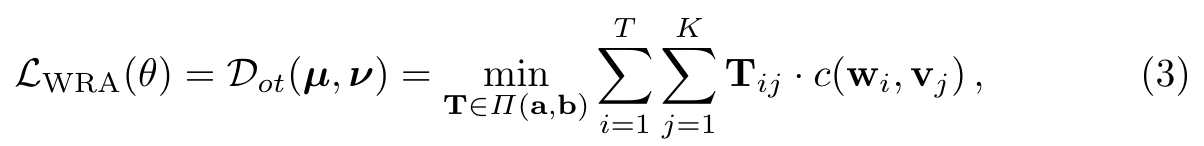where Π(a, b) = {T ∈ R^{T ×K}_{+} T1_m = a, T^T 1_n = b}, 1_n denotes an n-dimensional all-one vector, and c(w_i, v_j ) is the cost function evaluating the distance between w_i and v_j . In experiments, the cosine distance c(w_i, v_j ) = 1 − \frac{w^T_i v_j}{ w_i _2 v_j _2} is used. After solving T, the OT distance serves as the WRA loss that can be used to update the parameters θ. (p. 7)

1) Masked Region Feature Regression (MRFR) MRFR learns to regress the Transformer output of each masked region v(i) m to its visual features. (p. 7) 2) Masked Region Classification (MRC) MRC learns to predict the object semantic class for each masked region.Thus, we use the object detection output from Faster R-CNN, and take the detected object category (with the highest confidence score) as the label of the masked region (p. 7) 3) Masked Region Classification with KL-Divergence (MRC-kl) MRC takes the most likely object class from the object detection model as the hard label (w.p. 0 or 1), assuming the detected object class is the groundtruth label for the region. However, this may not be true, as no ground-truth label is available. Thus, in MRC-kl, we avoid this assumption by using soft label as supervision signal, which is the raw output from the detector (p. 7)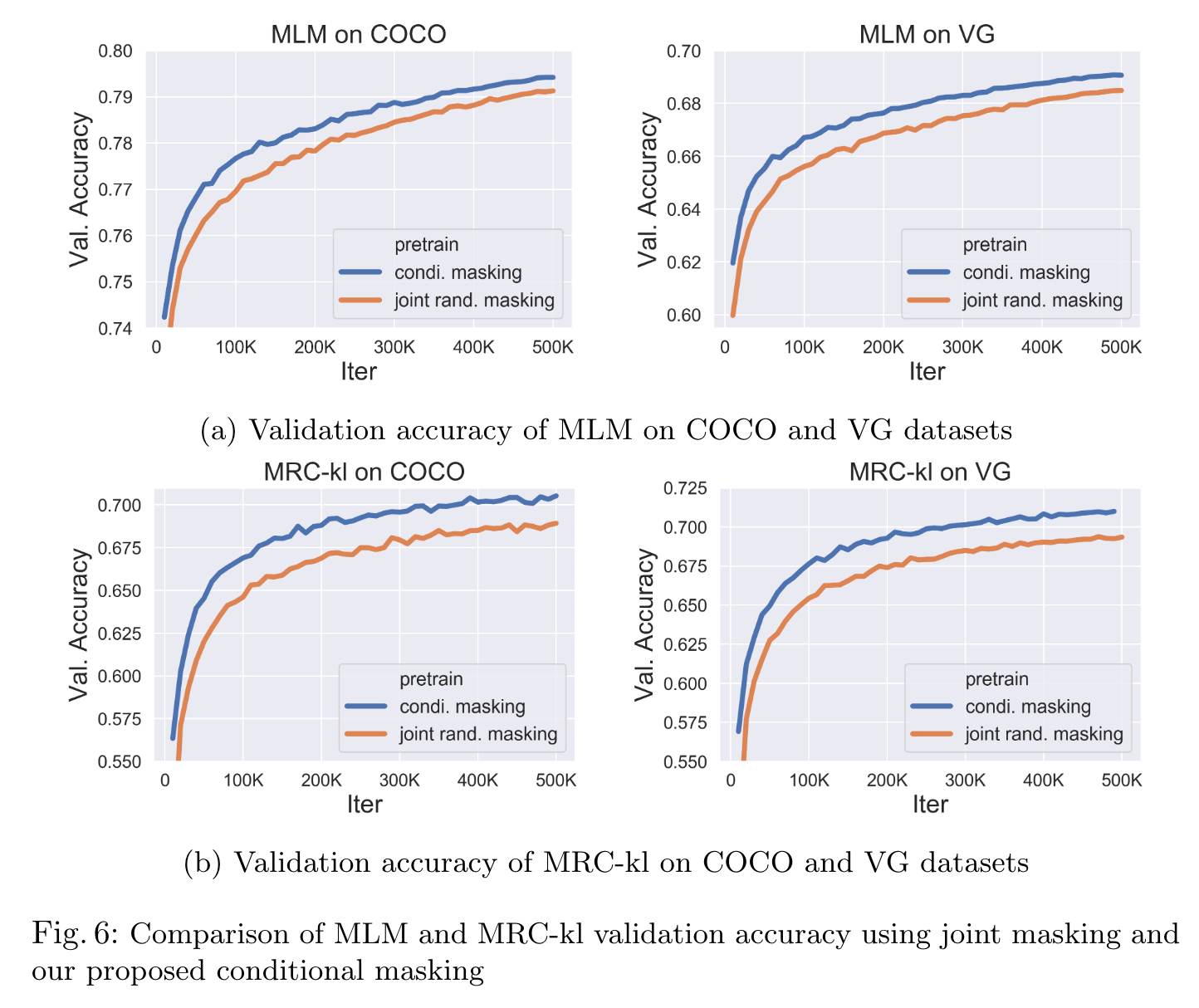WRA mostly benefits downstream tasks relying on region-level recognition: (p. 23)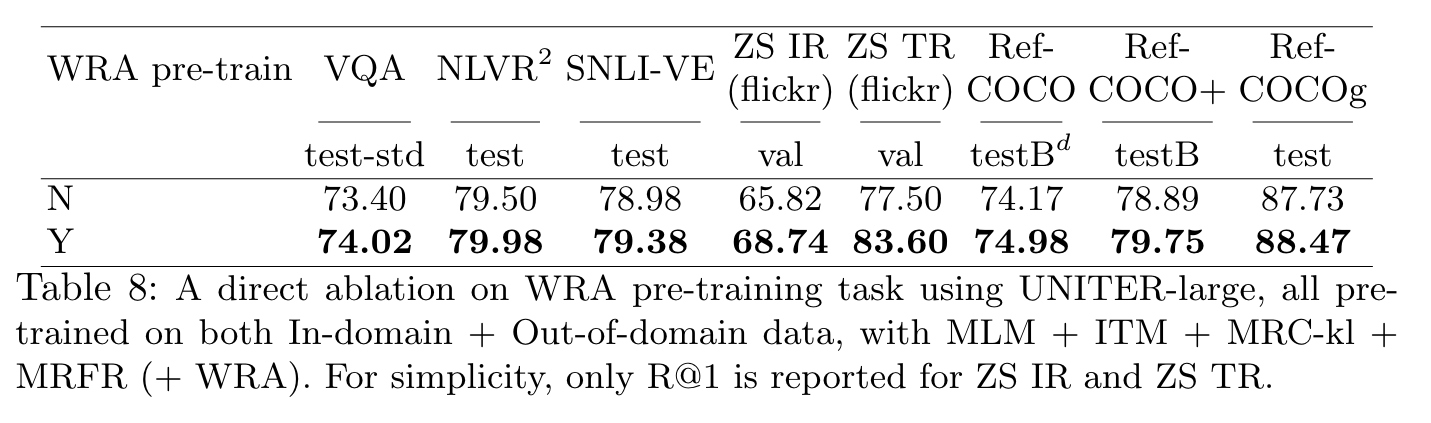## Pre-training Datasets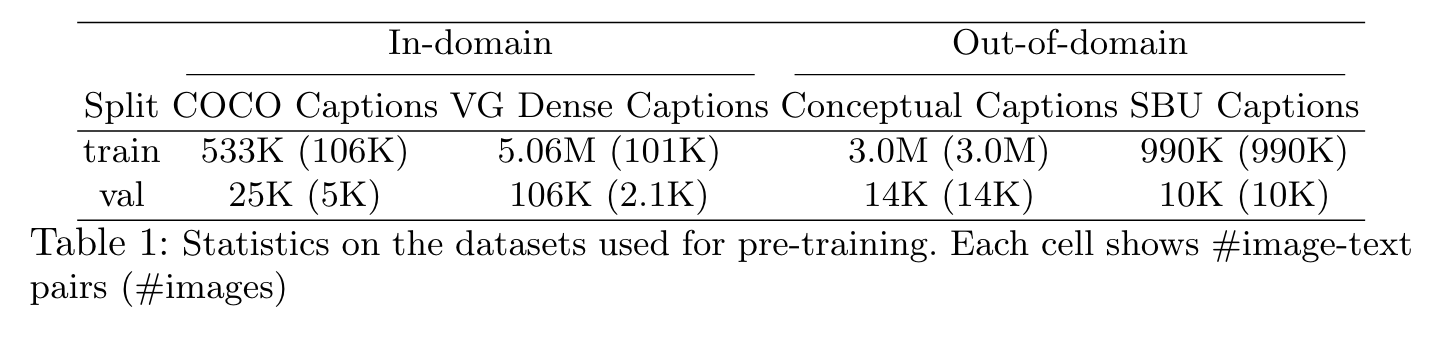# Experiments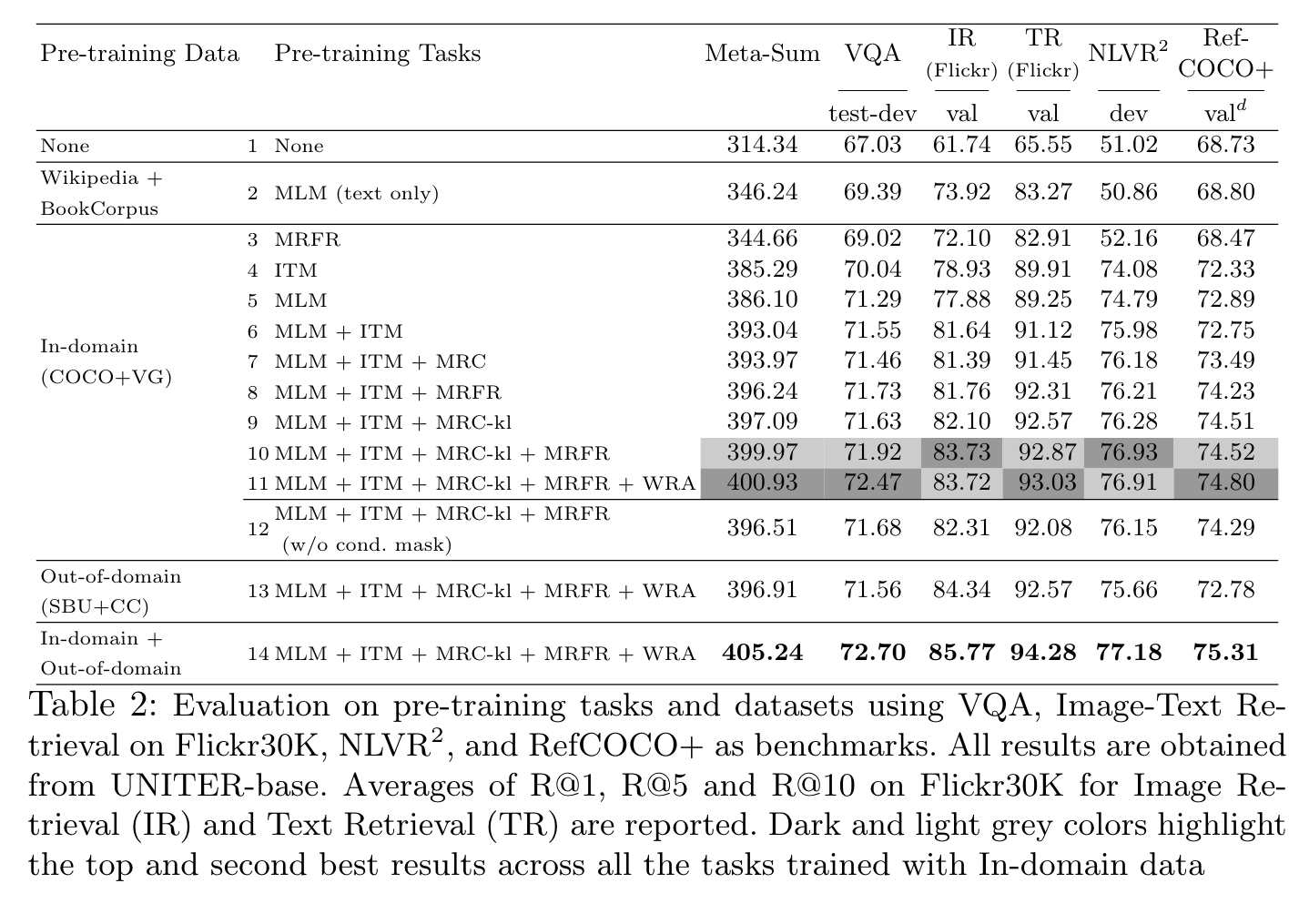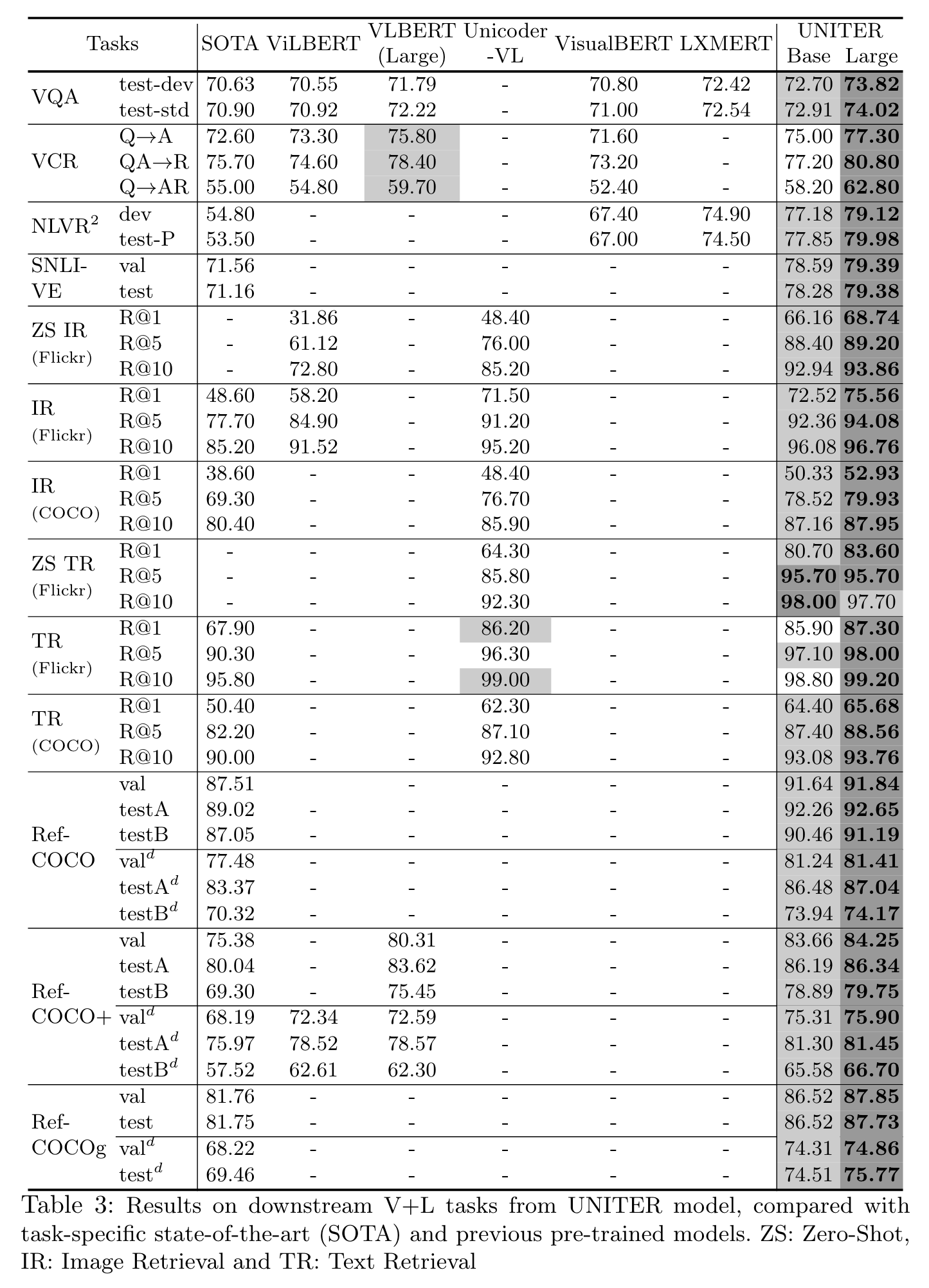## Visualization

1. Vertical: attention to special tokens [CLS] or [SEP];
2. Diagonal: attention to the token/region itself or preceding/following tokens/regions;
3. Vertical + Diagonal: mixture of vertical and diagonal;
4. Block: intra-modality attention, i.e., textual self-attention and visual selfattention;
5. Heterogeneous: diverse attentions that cannot be categorized and is highly dependent on actual input;
6. Reversed Block: inter-modality attention, i.e., text-to-image and image-totext attention. (p. 13)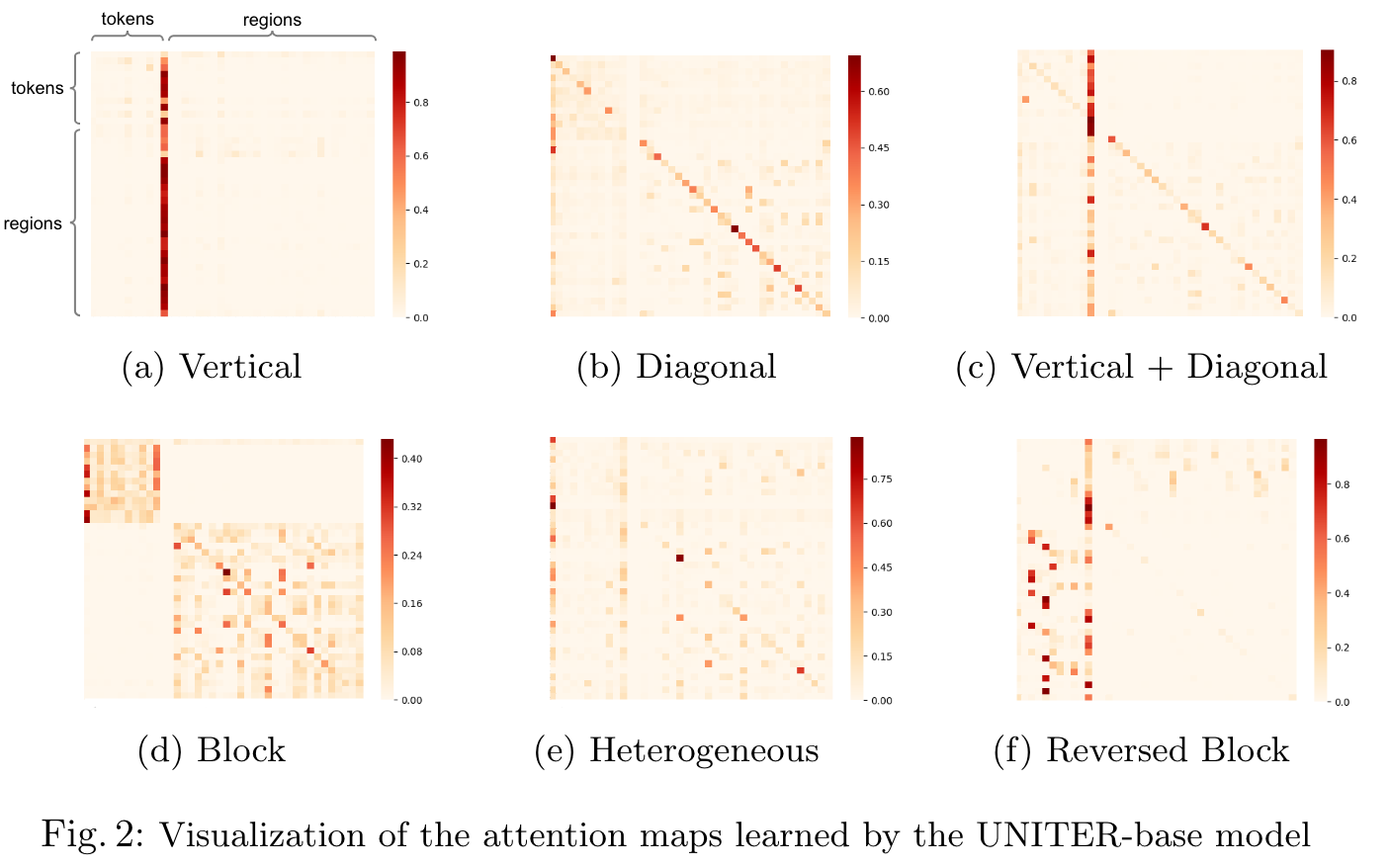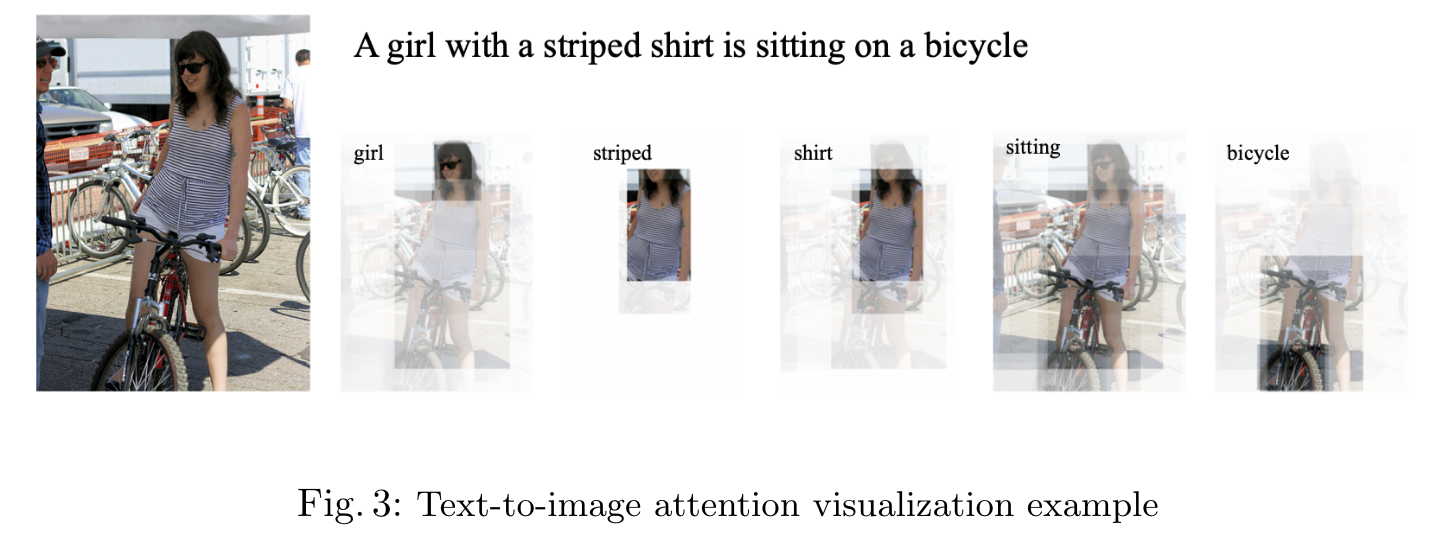# Ablation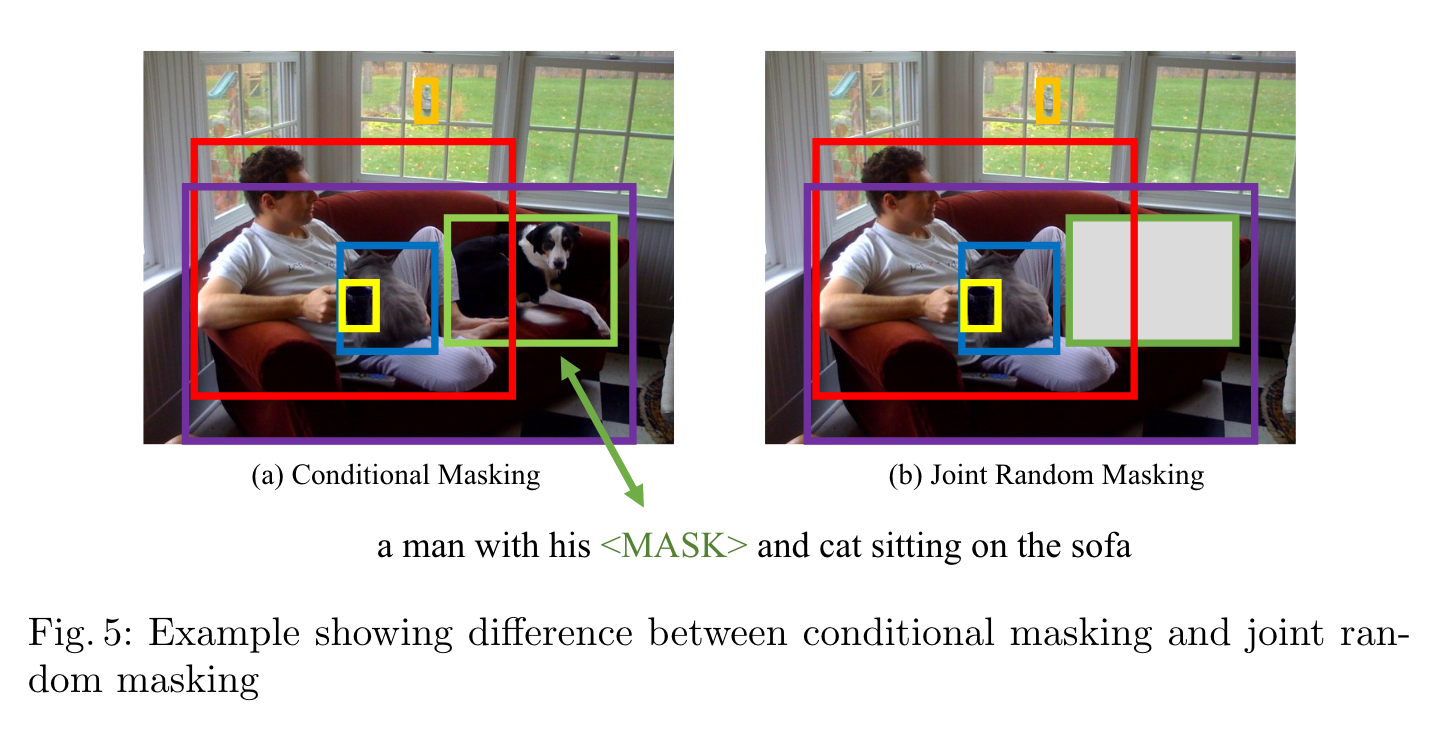Written on June 24, 2023